This book is archived and will be removed July 6, 2022. Please use the updated version.

Inductance

# 93 Mutual Inductance

### Learning Objectives

By the end of this section, you will be able to:

• Correlate two nearby circuits that carry time-varying currents with the emf induced in each circuit
• Describe examples in which mutual inductance may or may not be desirable

Inductance is the property of a device that tells us how effectively it induces an emf in another device. In other words, it is a physical quantity that expresses the effectiveness of a given device.

When two circuits carrying time-varying currents are close to one another, the magnetic flux through each circuit varies because of the changing current I in the other circuit. Consequently, an emf is induced in each circuit by the changing current in the other. This type of emf is therefore called a mutually induced emf, and the phenomenon that occurs is known as mutual inductance (M). As an example, let’s consider two tightly wound coils ((Figure)). Coils 1 and 2 have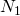and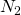turns and carry currents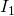and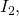respectively. The flux through a single turn of coil 2 produced by the magnetic field of the current in coil 1 is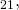whereas the flux through a single turn of coil 1 due to the magnetic field of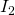is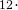Some of the magnetic field lines produced by the current in coil 1 pass through coil 2.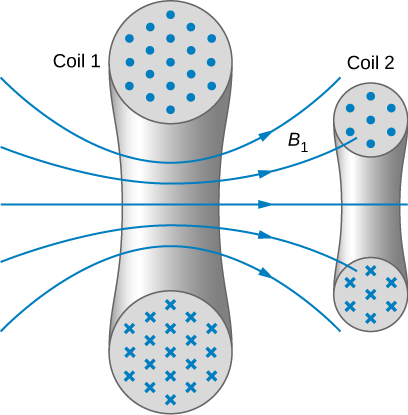The mutual inductance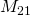of coil 2 with respect to coil 1 is the ratio of the flux through theturns of coil 2 produced by the magnetic field of the current in coil 1, divided by that current, that is,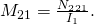Similarly, the mutual inductance of coil 1 with respect to coil 2 is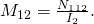Like capacitance, mutual inductance is a geometric quantity. It depends on the shapes and relative positions of the two coils, and it is independent of the currents in the coils. The SI unit for mutual inductance M is called the henry (H) in honor of Joseph Henry (1799–1878), an American scientist who discovered induced emf independently of Faraday. Thus, we have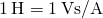. From (Figure) and (Figure), we can show that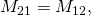so we usually drop the subscripts associated with mutual inductance and write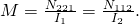The emf developed in either coil is found by combining Faraday’s law and the definition of mutual inductance. Since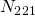is the total flux through coil 2 due to, we obtain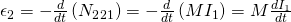where we have used the fact that M is a time-independent constant because the geometry is time-independent. Similarly, we have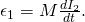In (Figure), we can see the significance of the earlier description of mutual inductance (M) as a geometric quantity. The value of M neatly encapsulates the physical properties of circuit elements and allows us to separate the physical layout of the circuit from the dynamic quantities, such as the emf and the current. (Figure) defines the mutual inductance in terms of properties in the circuit, whereas the previous definition of mutual inductance in (Figure) is defined in terms of the magnetic flux experienced, regardless of circuit elements. You should be careful when using (Figure) and (Figure) because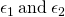do not necessarily represent the total emfs in the respective coils. Each coil can also have an emf induced in it because of its self-inductance (self-inductance will be discussed in more detail in a later section).

A large mutual inductance M may or may not be desirable. We want a transformer to have a large mutual inductance. But an appliance, such as an electric clothes dryer, can induce a dangerous emf on its metal case if the mutual inductance between its coils and the case is large. One way to reduce mutual inductance is to counter-wind coils to cancel the magnetic field produced ((Figure)).

The heating coils of an electric clothes dryer can be counter-wound so that their magnetic fields cancel one another, greatly reducing the mutual inductance with the case of the dryer.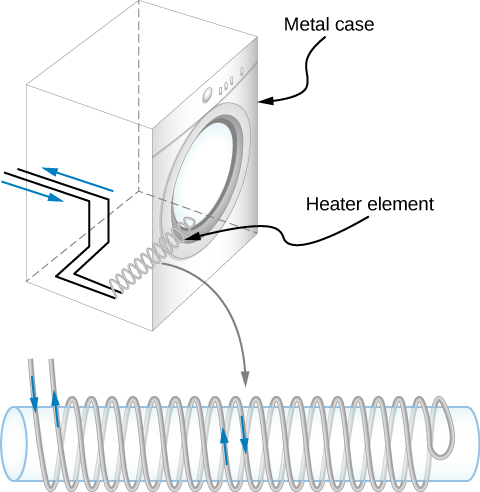Digital signal processing is another example in which mutual inductance is reduced by counter-winding coils. The rapid on/off emf representing 1s and 0s in a digital circuit creates a complex time-dependent magnetic field. An emf can be generated in neighboring conductors. If that conductor is also carrying a digital signal, the induced emf may be large enough to switch 1s and 0s, with consequences ranging from inconvenient to disastrous.

Mutual Inductance(Figure) shows a coil ofturns and radius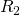surrounding a long solenoid of length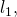radius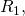andturns. (a) What is the mutual inductance of the two coils? (b) If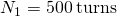,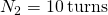,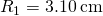,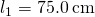, and the current in the solenoid is changing at a rate of 200 A/s, what is the emf induced in the surrounding coil?

A solenoid surrounded by a coil.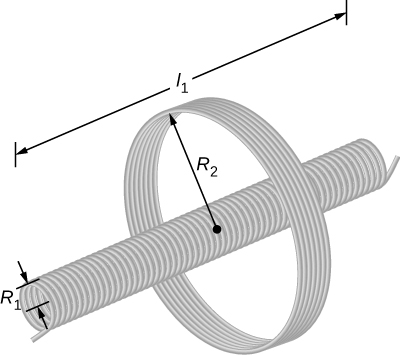Strategy There is no magnetic field outside the solenoid, and the field inside has magnitude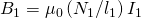and is directed parallel to the solenoid’s axis. We can use this magnetic field to find the magnetic flux through the surrounding coil and then use this flux to calculate the mutual inductance for part (a), using (Figure). We solve part (b) by calculating the mutual inductance from the given quantities and using (Figure) to calculate the induced emf.

Solution

1. The magnetic flux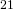through the surrounding coil is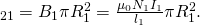Now from (Figure), the mutual inductance is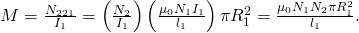2. Using the previous expression and the given values, the mutual inductance is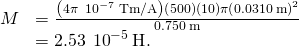Thus, from (Figure), the emf induced in the surrounding coil is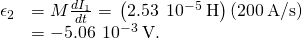Significance Notice that M in part (a) is independent of the radiusof the surrounding coil because the solenoid’s magnetic field is confined to its interior. In principle, we can also calculate M by finding the magnetic flux through the solenoid produced by the current in the surrounding coil. This approach is much more difficult because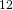is so complicated. However, since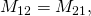we do know the result of this calculation.

Check Your Understanding A current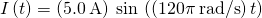flows through the solenoid of part (b) of (Figure). What is the maximum emf induced in the surrounding coil?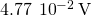### Summary

• Inductance is the property of a device that expresses how effectively it induces an emf in another device.
• Mutual inductance is the effect of two devices inducing emfs in each other.
• A change in current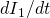in one circuit induces an emf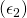in the second: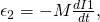where M is defined to be the mutual inductance between the two circuits and the minus sign is due to Lenz’s law.

• Symmetrically, a change in current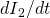through the second circuit induces an emf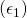in the first: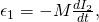where M is the same mutual inductance as in the reverse process.

### Conceptual Questions

Show that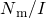and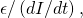which are both expressions for self-inductance, have the same units.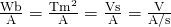A 10-H inductor carries a current of 20 A. Describe how a 50-V emf can be induced across it.

The ignition circuit of an automobile is powered by a 12-V battery. How are we able to generate large voltages with this power source?

The induced current from the 12-V battery goes through an inductor, generating a large voltage.

When the current through a large inductor is interrupted with a switch, an arc appears across the open terminals of the switch. Explain.

### Problems

When the current in one coil changes at a rate of 5.6 A/s, an emf of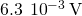is induced in a second, nearby coil. What is the mutual inductance of the two coils?

An emf of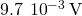is induced in a coil while the current in a nearby coil is decreasing at a rate of 2.7 A/s. What is the mutual inductance of the two coils?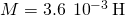Two coils close to each other have a mutual inductance of 32 mH. If the current in one coil decays according to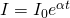, where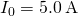and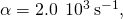what is the emf induced in the second coil immediately after the current starts to decay? At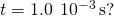A coil of 40 turns is wrapped around a long solenoid of cross-sectional areaThe solenoid is 0.50 m long and has 500 turns. (a) What is the mutual inductance of this system? (b) The outer coil is replaced by a coil of 40 turns whose radius is three times that of the solenoid. What is the mutual inductance of this configuration?

a.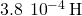; b.A 600-turn solenoid is 0.55 m long and 4.2 cm in diameter. Inside the solenoid, a small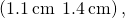single-turn rectangular coil is fixed in place with its face perpendicular to the long axis of the solenoid. What is the mutual inductance of this system?

A toroidal coil has a mean radius of 16 cm and a cross-sectional area of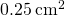; it is wound uniformly with 1000 turns. A second toroidal coil of 750 turns is wound uniformly over the first coil. Ignoring the variation of the magnetic field within a toroid, determine the mutual inductance of the two coils.A solenoid ofturns has length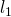and radiusand a second smaller solenoid ofturns has length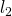and radius. The smaller solenoid is placed completely inside the larger solenoid so that their long axes coincide. What is the mutual inductance of the two solenoids?

### Glossary

henry (H)
unit of inductance,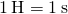; it is also expressed as a volt second per ampere
inductance
property of a device that tells how effectively it induces an emf in another device
mutual inductance
geometric quantity that expresses how effective two devices are at inducing emfs in one another